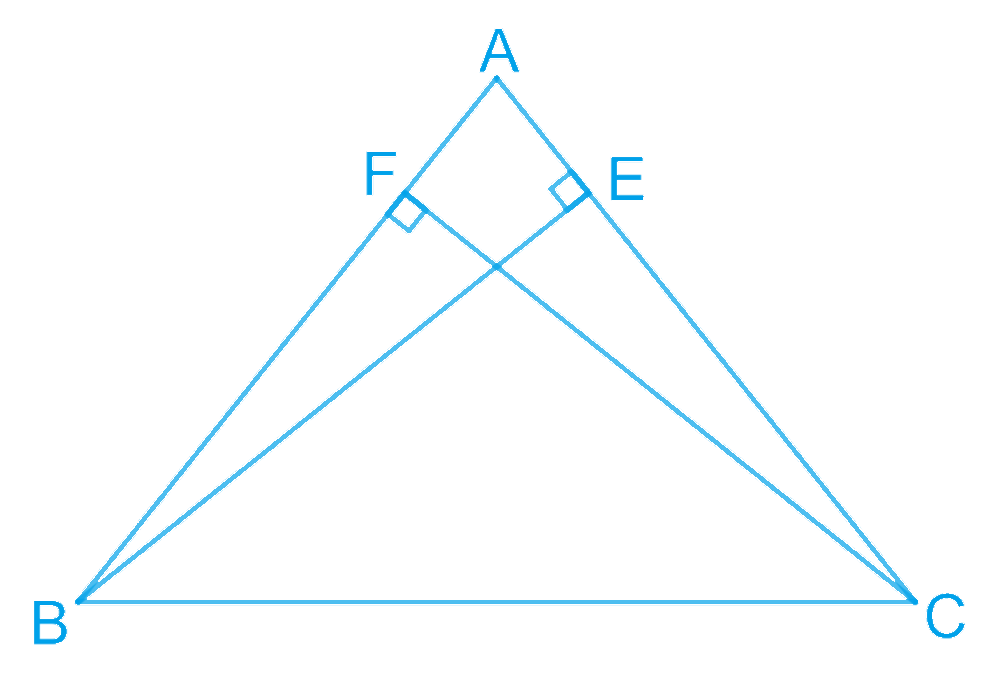# Ex.7.3 Q4 Triangles Solution - NCERT Maths Class 9

Go back to  'Ex.7.3'

## Question

$$BE$$ and $$CF$$ are two equal altitudes of a triangle $$ABC$$. Using RHS congruence rule, prove that the triangle $$ABC$$ is isosceles.

Video Solution
Triangles
Ex 7.3 | Question 4

## Text Solution

What is Known?

$$BE$$ and $$CF$$ are two equal altitudes of a triangle $$ABC.$$

To prove:

Triangle $$ABC$$ is isosceles by using RHS congruence rule.

Reasoning:

We can show triangles $$BEC$$ and $$CFB$$ congruent by using RHS congruency and then we can say corresponding parts of congruent triangles are equal to prove the required result.

Steps:In $$\Delta BEC$$ and $$\Delta CFB$$,

\begin{align} \angle BEC & = \angle CFB\;(\text{Each }90^{\circ})\\ BC &= CB\;(\text{Common})\\ BE &= CF\;(\text{Given})\\ \therefore \Delta BEC &\cong \Delta CFB\\ &\text{(By RHS congruency)} \end{align}

$$\therefore \angle BCE = \angle CBF (\text{By }CPCT)$$

$$\therefore AB = AC$$ (Sides opposite to equal angles of a triangle are equal) Hence, $$\Delta ABC$$ is isosceles.

Video Solution
Triangles
Ex 7.3 | Question 4

Learn from the best math teachers and top your exams

• Live one on one classroom and doubt clearing
• Practice worksheets in and after class for conceptual clarity
• Personalized curriculum to keep up with school